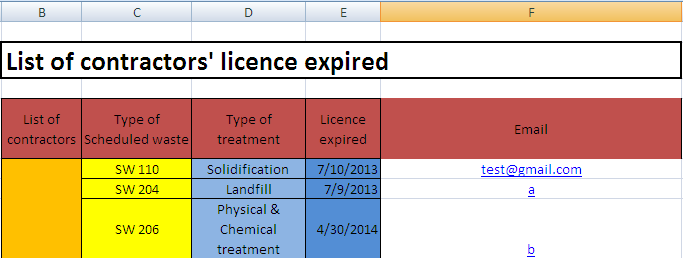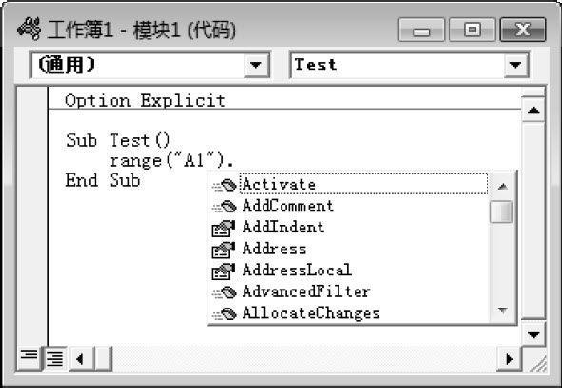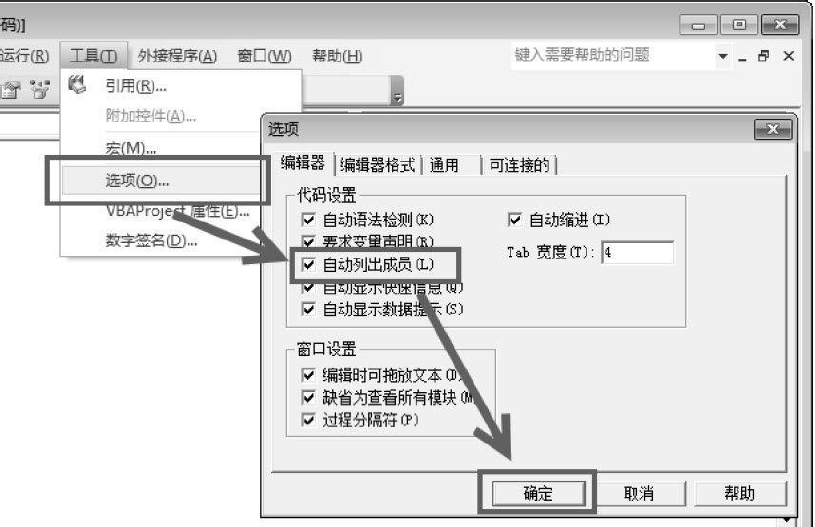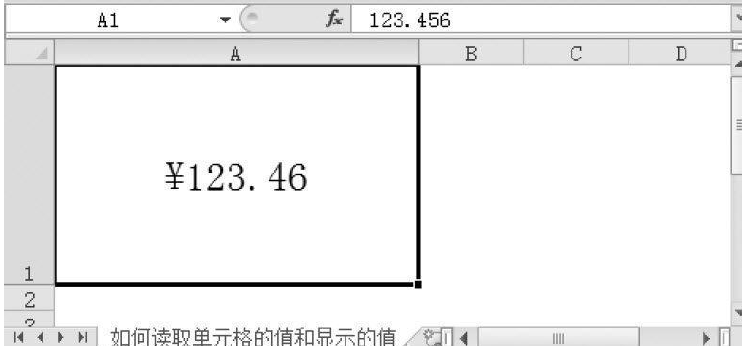• 二、使用VBA的SpecialCells 方法 该函数和下面Excel面板功能相通： 其使用格式为：expression.SpecialCells(Type, Value) expression 是必需的，返回一个有效的对象。 type也是必需的，在打出括号之后会有提示。 ...
• Sub 宏1() Dim M As String Dim arrResult() As ... arrResult = VBA.Split(M, "-") Cells(i, 2).Interior.Color = RGB(CInt(arrResult(0)), CInt(arrResult(1)), CInt(arrResult(2))) 'For i = LBound(...

Sub 宏1()

Dim M  As String
Dim arrResult() As String

For i = 1 To 365

M = Cells(i, 1)
arrResult = VBA.Split(M, "-")
Cells(i, 2).Interior.Color = RGB(CInt(arrResult(0)), CInt(arrResult(1)), CInt(arrResult(2)))

'For i = LBound(arrResult) To UBound(arrResult)
'   Debug.Print Trim(arrResult(i))

' Cells(i, 1).Interior.ColorIndex = i
Next i

End Sub

展开全文vba
• 获取代码如下 ... [a6] = Target.Address(0, 0) '获取选中单元格名称（字符名称 例如 C5 ) [a7] = ActiveCell.Cells.Row '获取选中单元格的行编号 数值 [a8] = ActiveCell.Cells.Column '获取选中单元.

获取代码如下

Sub Worksheet_SelectionChange(ByVal Target As Range)

[a5] = ActiveCell.Cells   '获取选中单元格内容
[a6] = Target.Address(0, 0) '获取选中单元格名称（字符名称 例如  C5 )
[a7] = ActiveCell.Cells.Row '获取选中单元格的行编号  数值
[a8] = ActiveCell.Cells.Column '获取选中单元格的列编号  数值

End Sub

下面是复制来的内容

excel vba单元格引用的三种方式
vba单元格引用方法有几种，比如要引用A1单元格，
写法可以这样的：range("A1")或者cells(1,1)或者[A1]。

excel vba单元格引用写法一：

Cells(1, 1) = "abc123"

excel vba单元格引用写法二：

1.Range("单个单元格")

比如，Range("A1") = "abc123"
注意：单元格地址要加双引号，而且双引号的输入必须是在半角状态下输入的，否则会出现错误。单元格地址不区分相对引用和绝对引用的，无论使用Range("A1")、Range("$A1")、Range("$A$1")还是Range("A$1")，都是引用同一个单元格。

2. Range("单元格区域")
在VBA数组中，经常遇到arr=Range("A1:D10")这种数组赋予方式，而里面出现了Range("单元格区域")的这种单元格区域引用。
"A1:D10"代表一个单元格区域的引用，其中A1是该区域的左上角上面的单元格，而D10则是该区域右下角下面的单元格。

又如：Range("B2:E6")(3).Select，这句代码的意思是选取B2：E6区域中的第三个单元格，即D2，而单元格的区域的读取一般是从左到右，然后再从上到下进行读取的，故该区域的前3个单元格分别是B2、C2、D2，而第3个单元格就是为D2。

excel vba单元格引用写法三：[A1]

比如， [A1] = "abc123"
另外，[A1:D10].Select，表示选取A1：D10区域。

by anlog

2021年6月4日

展开全文vba获取选中单元格
• task to send email from excel.Below is the example column. How I can get the email value from the loop?ThanksHere is my code :Dim rng As RangeDim row As RangeDim cell As RangeDim mydate As DateDim myD...task to send email from excel.

Below is the example column. How I can get the email value from the loop?

ThanksHere is my code :

Dim rng As Range

Dim row As Range

Dim cell As Range

Dim mydate As Date

Dim myDiff As Long

Set rng = Range("E5:E17")

Set rng2 = Range("F5:F17")

Dim cell2 As Range

Dim i As Integer

i = 5

For Each row In rng.Rows

For Each cell In row.Cells

MsgBox cell.Value

mydate = cell.Value

myDiff = DateDiff("d", Date, mydate)

Set rng2 = Range("F" & i)

For Each cell2 In rng2

MsgBox cell2.Value

Next cell2

If myDiff > -8 And myDiff < 8 Then

aEmail.Send

End If

i = i + 1

Next cell

Next row

解决方案

I think you need this ..

Dim n as Integer

For n = 5 to 17

msgbox cells(n,3) '--> sched waste

msgbox cells(n,4) '--> type of treatm

msgbox format(cells(n,5),"dd/MM/yyyy") '--> Lic exp

msgbox cells(n,6) '--> email col

Next

展开全文• 单元格对象的Value属性为单元格实际存储的，当单元格中存有公式时，Value属性为公式运行后的结果。当需要表示某个对象的属性时，可在对象与属性之间运用点(．)运算符。如获取A1单元格，可以用： Range(...

目录

单元格对象的Value属性

单元格对象的Text属性

自动列出成员

单元格的数字格式属性

示例：

单元格对象的Value属性

单元格对象的Value属性为单元格实际存储的值，当单元格中存有公式时，Value属性为公式运行后的结果值。当需要表示某个对象的属性时，可在对象与属性之间运用点(．)运算符。如获取A1单元格的值，可以用：

Range("A1").Value

Value属性为单元格对象的默认属性，当需要获取某个对象的默认属性时，可以直接用对象表示。
非特殊情况下，不建议开发者省略对象的默认属性，否则可能造成程序出错。当某些对象的默认属性同样是一个对象时，若用Set语句获取其默认属性，VBA将会认为是获取该对象而非其默认属性。

单元格对象的Text属性

单元格对象的Text属性为其显示的值，即进行数字格式化后所显示的值。
由于用户可以对单元格进行数字格式的设置，因而Text属性和Value属性在大多数情况下是不相等的。

自动列出成员

在Excel中，大部分对象都拥有超过20个属性或方法，初学者通常无法记住所有的属性或方法。在编写程序时，VBE提供了非常快捷的成员提示。当某个对象的类型确定后，开发者只需写至点运算符时，VBE会自动列出该对象所有的属性或方法，如图所示。可以使用方向键选择某个属性或方法，然后按【Tab】键确认输入，VBE将会自动填充该对象的属性或方法。开发者可参照如图所示的步骤开启或关闭成员列表提示。单元格的数字格式属性

表示单元格对象的数字格式有两个属性：NumberFormat和NumberFormatLocal。这两个属性都表示单元格对象的数字格式。不同的是，NumberFormat表示的是通用的数字格式，而NumberFormatLocal则表示本地的数字格式，该数字格式是由控制面板的区域设定而转换的数字格式。通常情况下，若需要通过VBA设置单元格格式，可采用NumberFormat属性进行赋值。

示例：

如图所示，A1单元格设置了“货币”的数字格式，其单元格实际存储的值和显示的值是不同的。如何利用VBA分别读取该单元格的值以及显示的值？展开全文• Sub 把单元格值赋给数组() Dim ary() Dim i, j, k, rowall, columnall, rownum As Integer rownum = InputBox("输入标题行所占行数") rowall = Cells(Rows.Count, 1).End(xlUp).Row columnall = Cells(1, Columns....vba
• VBA示例之 选择指定范围内使用了填充颜色的单元格，供初学者参考，大牛勿进~~~~~~~
• VBA记录更改之前的，判断是否执行其他VBA操作等，源码在Sheet1
• VBA操作Excel简介一、VBA读写Excel文件 一、VBA读写Excel文件vba excel
• 通过上一节, 我们己经可以掌握进入vba的编辑页面,可以愉快的代码了.  接下来,给大家看看怎么取得表格里一个单元格.因为只有拿了这个,你才能对它进行各种改造 如下图: 单元格 A1 里有一个: 点点...excel vba
• VBA中实现Excel表格单元格复制，可以根据需要自行定制要复制的内容。包括、格式、单元格宽度、背景色等
• VBA示例之 在特定的区域内查找文本,返回值是包含查找文本的单元格，供初学者参考，大牛勿进~~~~~~~
• How to get the value of a merged cell of an excel having range address like "$B$4:$B$11" in vba解决方案Even if it is really discouraged to use merge cells in Excel (use Center Across Selection for ...
• 如何对多个不相邻的单元格批量赋予同样的？我是小白，按照自己的逻辑这样了，可是不行：Sub ID赋值和日期赋值()Sheet3.Range ("C1")Sheet3.Range ("M1")Sheet3.Range ("W1")Sheet3.Range ("AG1")Sheet3.Range (...
• 今天还是讲讲单元格操作 一、删除空行 如果是上面的表格要删除空行的话，我们想到的是先删掉第1行，再第2行，再第3行……但是对于程序来说，删完第1行后，第2行就变成了第1行后面就会出错，所以我们先从后面...
• VBA编程经常要读取、写入单元格内容，所以总结这么一篇笔记，我只是把最常用到的功能列举出来。具体用法有疑惑可以百度。 ThisWorkbook：当前工作簿，这个跟VBA代码编写位置有关系，如下图所示： ActiveWorkbook：...vba
• excel VBA实现两个excel文件所有worksheet单元格做对比，并找出差异，差异进行着重显示，对于数据量非常在和对数据要求高的场景会大大缩减人工对比的时间
• wi).Select '如果需要向下粘贴单元格，注释掉上一行，并取消注释下面一行 'ActiveSheet.Cells(ro + hi, co).Select Dim m As Integer m = 0 m = InputBox("请输入您需要拷贝的数量", "请输入") While m > 0 If ...
• 一、提示用户输入 Sub SetValue() Sheets("sheet3").Range("a1") = InputBox("输入一个数字") End Sub 运行后就会弹出个警告框，提示用户输入内容，输入后就会在A1单元格显示了，但是也存在一个问题，就是取消...
• VBA：Excel选中单元格后，相同高亮显示 首先是完整的函数代码 Private Sub Worksheet_SelectionChange(ByVal Target As Range) If Target.Count > 1 Then Exit Sub Dim rng As Range If Target.Count = 1 And ...VBA
• VBA源代码，根据某列中的最大，拿该行的行号。此代码为取出若干小区的信息，7*24小时，拿某列最大的行号，精简成7行
• VBA实现Excel单元格下拉框复选demo，需要结合VB代码实现，demo很详细
• 直接上代码在sheet1里面，顺便修改sheet1为Sht1 Sub Image_Change() Dim x%, y%, w%, h%, iPath\$ With ActiveSheet.Shapes.Range(Array("图片")) x = .Left '记录坐标点 y = .Top '记录坐标点 ...EXCEL VBA
• 前言：最近在做一个地址标准化的竞赛，里面包括了地址提取、分词、清洗、匹配等一系列动作，等这次竞赛结束后会专门一篇文章分享源码。 之所以要做标题的事情，是因为用了这么多年的Excel，还是习惯Excel的界面，...vba
• ArrAction(), ArrFaceID(), ArrToolTip()Dim i As IntegerArrCaption = Array('查找', '功能二', '功能三')ArrAction = Array('查找选定单元格内容', ...
• 整个表格的内容由填写序号的单元格引发变化，每变化一次另存为pdf，并其中一个单元格作为pdf的文件名 一、错误写法 Sub 另存并重命名PDF() Dim i As Integer Dim n As String For i = 1 To 10 Sheets(...vba excel
• vba选中的单元格到剪贴板If you want to share a smallpart of your Excel worksheet but don’t want to send an Excel file, you can copy part of the worksheet to the clipboard as a picture instead, which ...excel python 深度学习 vba 图像识别
• 检查 单元格中文 Sub test() For i = 2 To 358010 If (Len(Cells(i, 2)) < LenB(StrConv(Cells(i, 2), vbFromUnicode))) Then Cells(i, 3) = 1 End If Next i End Sub 检查单元格字符串 Sub test() For i...
• 最近因某项目的客户需求，需要使用vba，监听某工作簿的某工作表内单元格是否发生变化（在已有上手动修改或者在空白处添加新），如果有变化设置变化后的单元格的字体为红色。 作为初学vba的小白，在网上查了...vba...